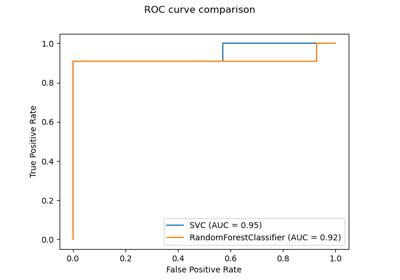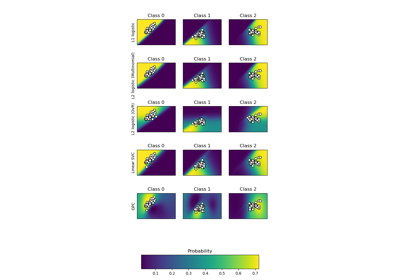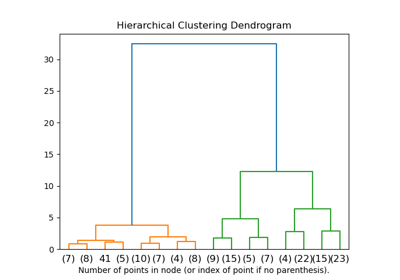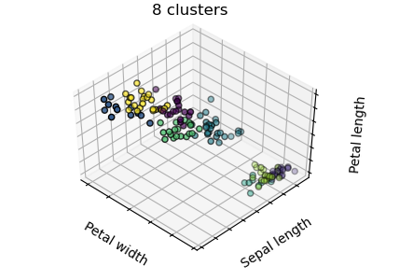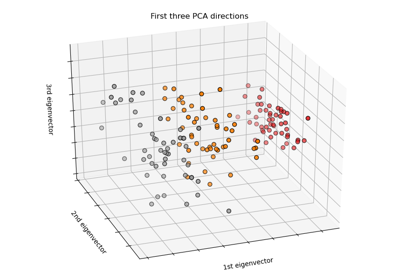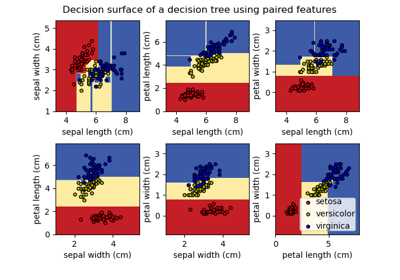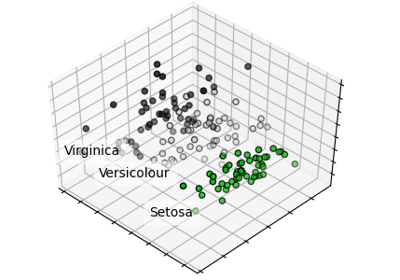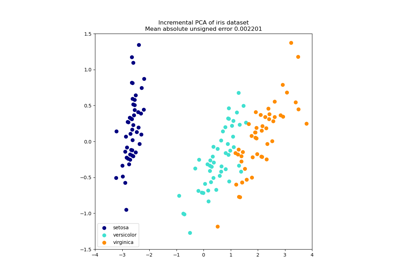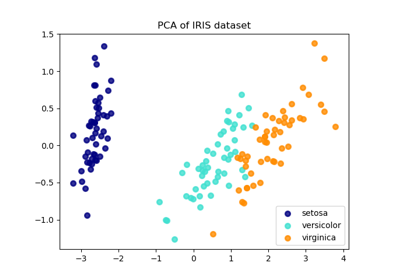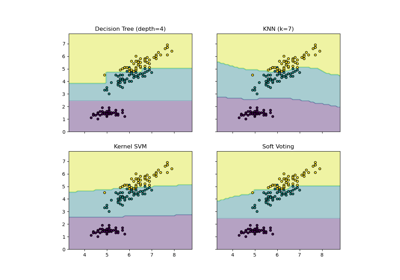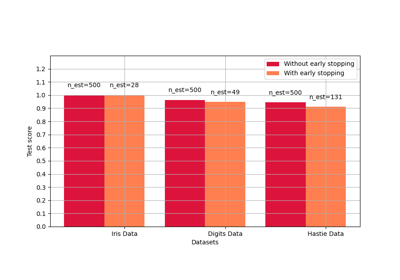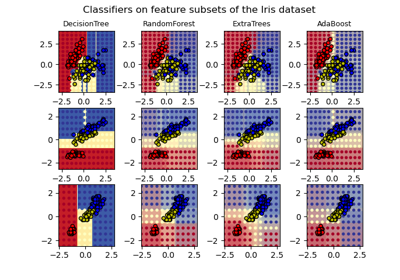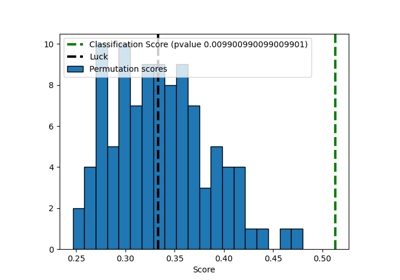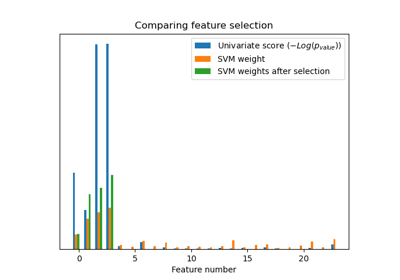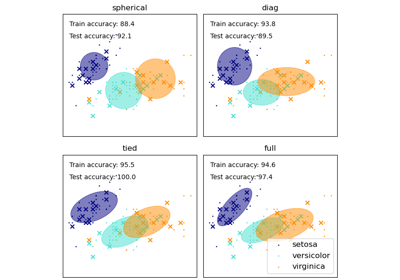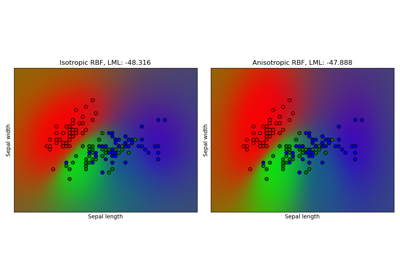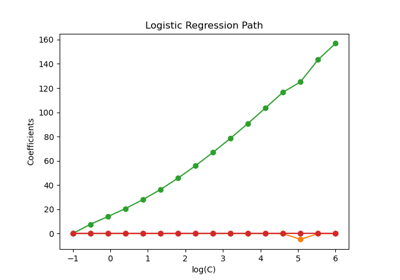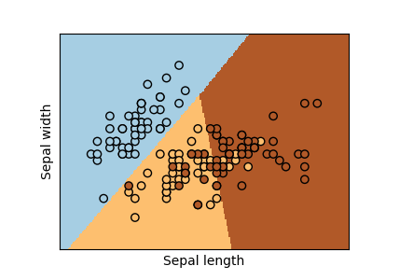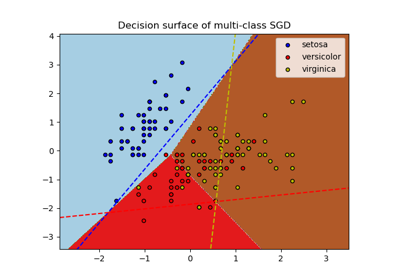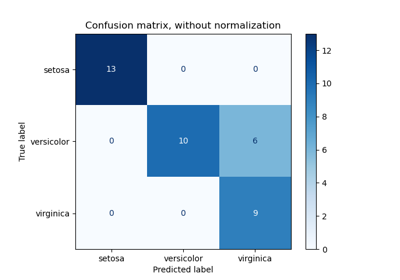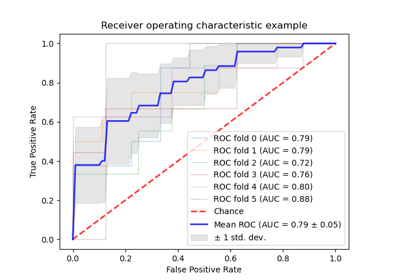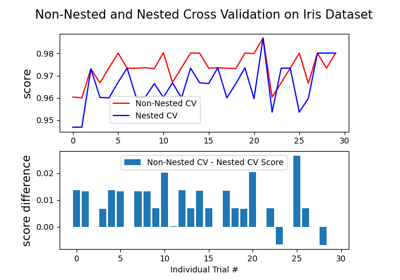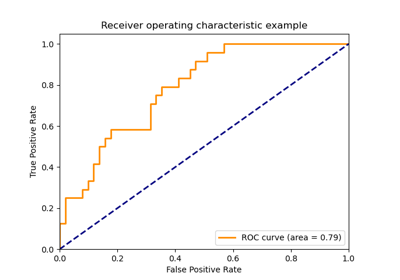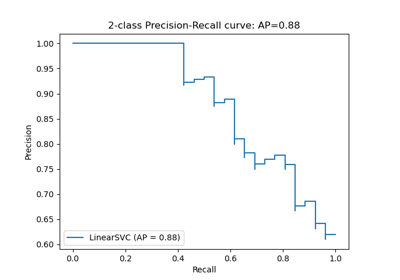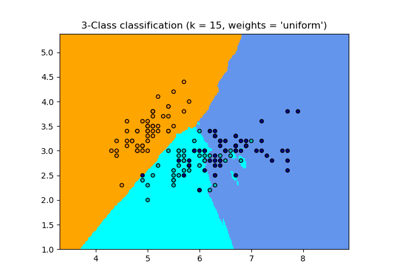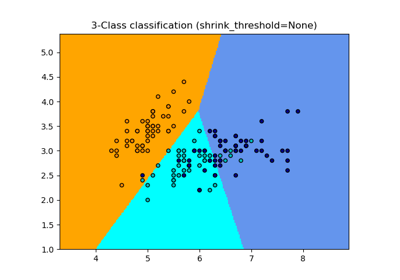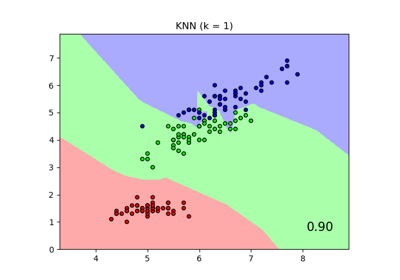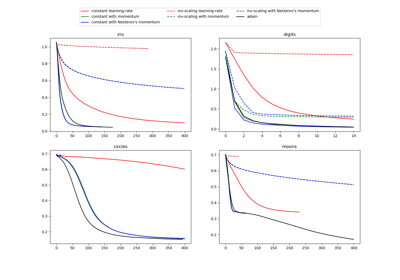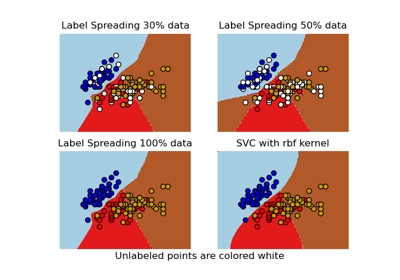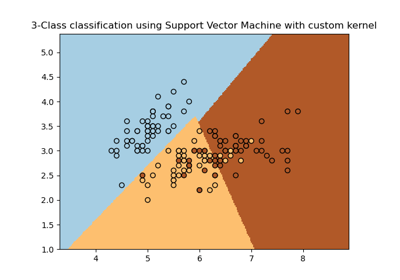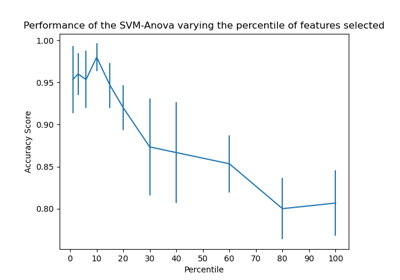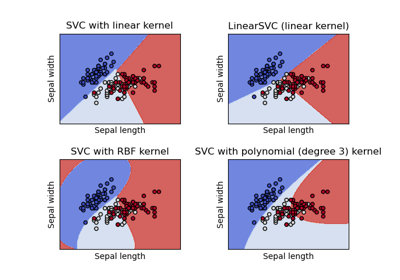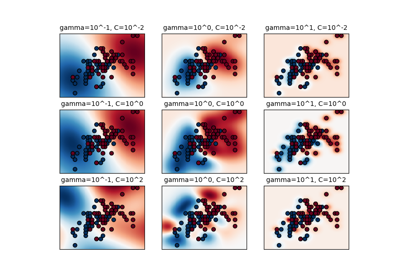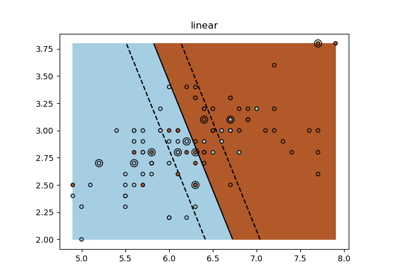# sklearn.datasets.load_iris¶

sklearn.datasets.load_iris(*, return_X_y=False, as_frame=False)[source]

Load and return the iris dataset (classification).

The iris dataset is a classic and very easy multi-class classification dataset.

 Classes 3 Samples per class 50 Samples total 150 Dimensionality 4 Features real, positive

Read more in the User Guide.

Parameters
return_X_ybool, default=False.

If True, returns (data, target) instead of a Bunch object. See below for more information about the data and target object.

New in version 0.18.

as_framebool, default=False

If True, the data is a pandas DataFrame including columns with appropriate dtypes (numeric). The target is a pandas DataFrame or Series depending on the number of target columns. If return_X_y is True, then (data, target) will be pandas DataFrames or Series as described below.

New in version 0.23.

Returns
dataBunch

Dictionary-like object, with the following attributes.

data{ndarray, dataframe} of shape (150, 4)

The data matrix. If as_frame=True, data will be a pandas DataFrame.

target: {ndarray, Series} of shape (150,)

The classification target. If as_frame=True, target will be a pandas Series.

feature_names: list

The names of the dataset columns.

target_names: list

The names of target classes.

frame: DataFrame of shape (150, 5)

Only present when as_frame=True. DataFrame with data and target.

New in version 0.23.

DESCR: str

The full description of the dataset.

filename: str

The path to the location of the data.

New in version 0.20.

(data, target)tuple if return_X_y is True

New in version 0.18.

Notes

Changed in version 0.20: Fixed two wrong data points according to Fisher’s paper. The new version is the same as in R, but not as in the UCI Machine Learning Repository.

Examples

Let’s say you are interested in the samples 10, 25, and 50, and want to know their class name.

>>> from sklearn.datasets import load_iris
>>> data.target[[10, 25, 50]]
array([0, 0, 1])
>>> list(data.target_names)
['setosa', 'versicolor', 'virginica']


## Examples using sklearn.datasets.load_iris¶